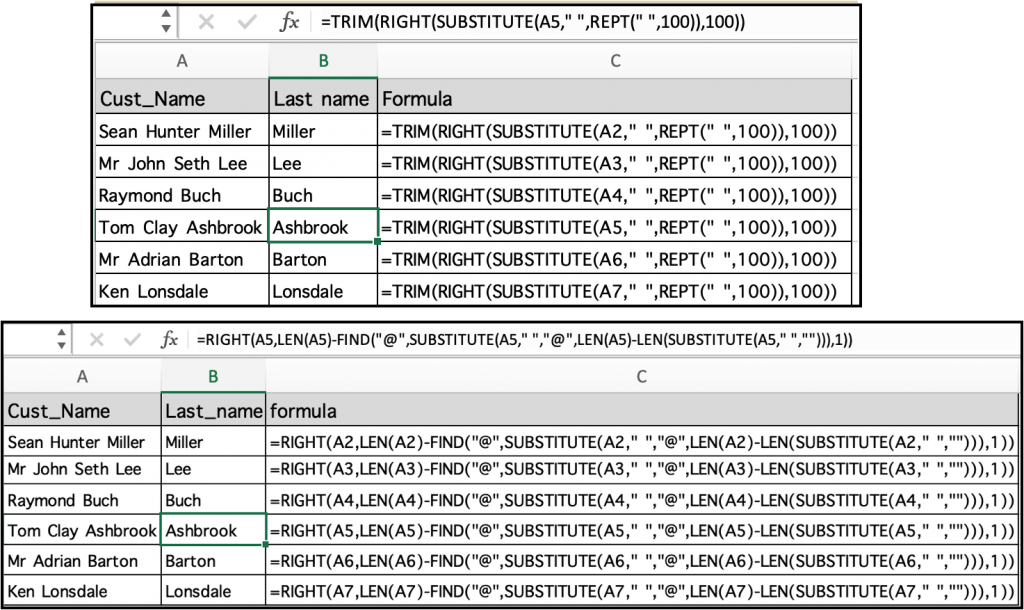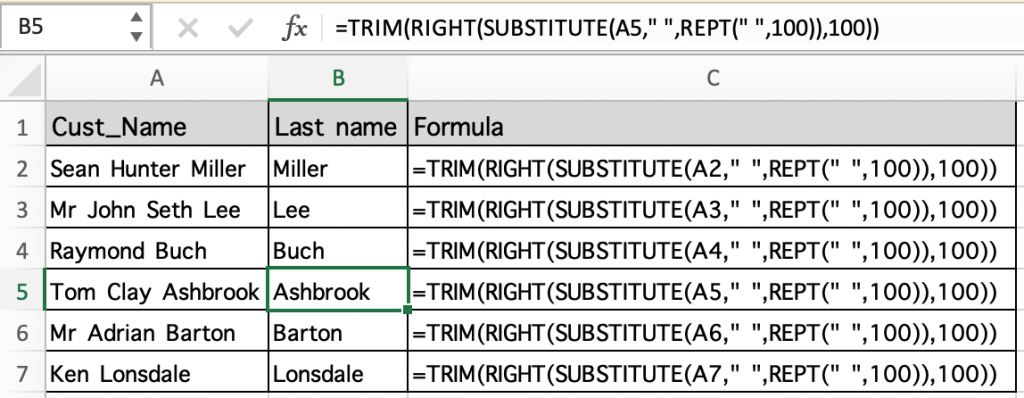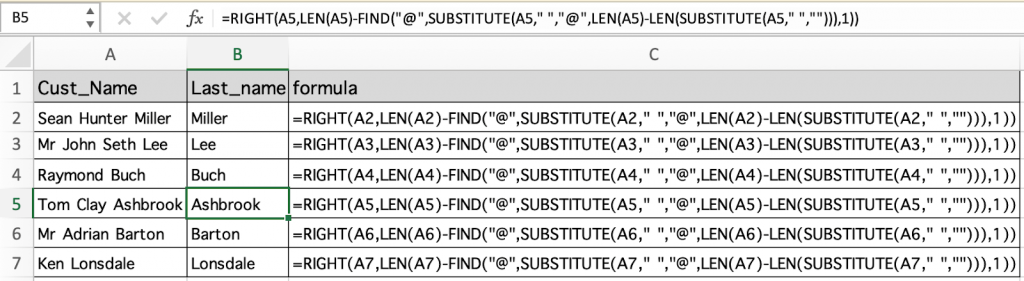# How to Extract last Word in ExcelIn this article, we will learn How to Extract last Word in Excel.

Scenario:

Working with text data in excel. Sometimes we come across  the problem of splitting words from the full text. For example splitting full names into first name and last name. Or extracting the names from the given email ids. For situations like these we use LEFT and RIGHT functions in excel along with some basic sense of how excel functions work. How to recognize where to separate the full name like Nedra Kleiber. Here a space character in between the first name and last name. So we direct excel to find the position of last space char in the full name and separate it into two using functions. Let's understand how the below given formula does it.

Last name formula in Excel

Here given a name value as text in the formula. First of all the find function gives the position of last space character and LEN function gives the length of full text. And the RIGHT function does rest to extract the word.

Last name formula using TRIM, RIGHT, SUBSTITUTE and REPT functions

 =TRIM(RIGHT(SUBSTITUTE(text," ",REPT(" ",100)),100))

text : full name

" " : Space character given in quotes. Or use char function

Last name formula using LEN, RIGHT, SUBSTITUTE and FIND functions

 =RIGHT(text,LEN(text)-FIND("@",SUBSTITUTE(text," ","@",LEN(text)-LEN(SUBSTITUTE(text," ",""))),1))

text : full name

" " : Space character given in quotes. Or use char function

"@" : here @ is used to replace space char. Where "@" is a lookup value, then use any other unique character.

Example :

All of these might be confusing to understand. Let's understand how to use the function using an example. Here we are given some sample names and we need to extract the last name only in another column. So first we start with a basic formula that is first name extraction. You can provide text values using text in quotes or by using cell reference which is explained here.

Use the formula:

 =TRIM(RIGHT(SUBSTITUTE(A2," ",REPT(" ",100)),100))

Copy and paste the formula to other cells using the keyboard shortcut Ctrl + D or dragging the right bottom corner box till the required cell.As you can see in the above image that only the last name is extracted from the full names independent of the number of spaces in the full name. Let's try another formula mentioned below to get the same result

Alternate method

Here we are given some sample names and we need to extract the last name only in another column using a different formula. So first we start with a basic formula that is first name extraction. You can provide text values using text in quotes or by using cell reference which is explained here.

Use the formula:

 =RIGHT(A2,LEN(A2)-FIND("@",SUBSTITUTE(A2," ","@",LEN(A2)-LEN(SUBSTITUTE(A2," ",""))),1))

Copy and paste the formula to other cells using the keyboard shortcut Ctrl + D or dragging the right bottom corner box till the required cell.As you can see in the above image that only the last name is extracted from the full names independent of the number of spaces in the full name.

You can use any of the two mentioned formulas to get the result. Use the one you are comfortable using the functions.

Here are all the observational notes using the formula in Excel
Notes :

1. Text values in formula be used with between quotes or using cell reference. Using cell reference in excel is the best common practice.
2. Use the =CHAR(32) function to get the space character in the formula.

Hope this article about How to Extract last Word in Excel is explanatory. Find more articles on calculating values and related Excel formulas here. If you liked our blogs, share it with your friends on Facebook. And also you can follow us on Twitter and Facebook. We would love to hear from you, do let us know how we can improve, complement or innovate our work and make it better for you. Write to us at info@exceltip.com.

Related Articles :

Data Validation in Excel : Data Validation is a tool used to restrict users to input value manually in cell or worksheet in Excel. It has a list of options to choose from.

Way to use Vlookup function in Data Validation : Restrict users to allow values from the lookup table using Data validation formula box in Excel. Formula box in data validation allows you to choose the type of restriction required.

Restrict Dates using Data Validation : Restrict user to allow dates from a given range in cell which lays within Excel date format in Excel.

How to give the error messages in Data Validation : Restrict users to customize input information in the worksheet and guide the input information through error messages under data validation in Excel.

Create Drop Down Lists in Excel using Data Validation : Restrict users to allow values from the drop down list using Data validation List option in Excel. List box in data validation allows you to choose the type of restriction required.

Popular Articles :